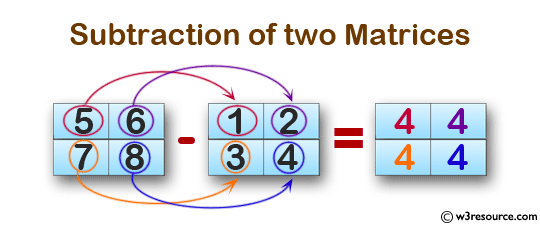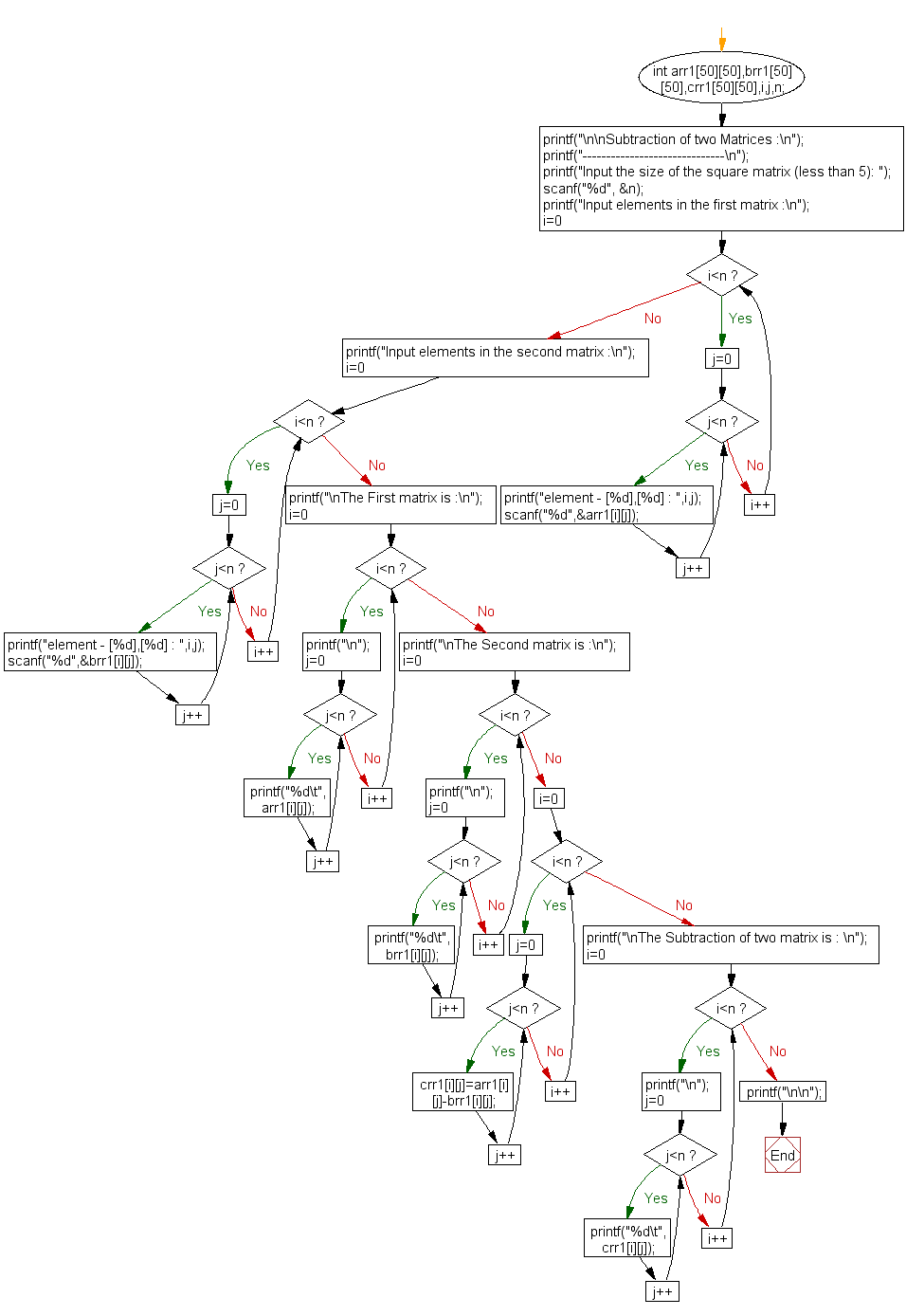﻿ C exercises: Subtraction of two Matrices - w3resource# C Exercises: Subtraction of two Matrices

## C Array: Exercise-20 with Solution

Write a program in C for subtraction of two Matrices.

Pictorial Presentation:Sample Solution:

C Code:

``````#include <stdio.h>

void main()
{
int arr1,brr1,crr1,i,j,n;

printf("\n\nSubtraction of two Matrices :\n");
printf("------------------------------\n");
printf("Input the size of the square matrix (less than 5): ");
scanf("%d", &n);

/* Stored values into the array*/
printf("Input elements in the first matrix :\n");
for(i=0;i<n;i++)
{
for(j=0;j<n;j++)
{
printf("element - [%d],[%d] : ",i,j);
scanf("%d",&arr1[i][j]);
}
}

printf("Input elements in the second matrix :\n");
for(i=0;i<n;i++)
{
for(j=0;j<n;j++)
{
printf("element - [%d],[%d] : ",i,j);
scanf("%d",&brr1[i][j]);
}
}
printf("\nThe First matrix is :\n");
for(i=0;i<n;i++)
{
printf("\n");
for(j=0;j<n;j++)
printf("%d\t",arr1[i][j]);
}

printf("\nThe Second matrix is :\n");
for(i=0;i<n;i++)
{
printf("\n");
for(j=0;j<n;j++)
printf("%d\t",brr1[i][j]);
}
/* calculate the subtraction of the matrix */
for(i=0;i<n;i++)
for(j=0;j<n;j++)
crr1[i][j]=arr1[i][j]-brr1[i][j];
printf("\nThe Subtraction of two matrix is : \n");
for(i=0;i<n;i++){
printf("\n");
for(j=0;j<n;j++)
printf("%d\t",crr1[i][j]);
}
printf("\n\n");
}
```
```

Sample Output:

```Subtraction of two Matrices :
------------------------------
Input the size of the square matrix (less than 5): 2
Input elements in the first matrix :
element - , : 5
element - , : 6
element - , : 7
element - , : 8
Input elements in the second matrix :
element - , : 1
element - , : 2
element - , : 3
element - , : 4

The First matrix is :
5       6
7       8
The Second matrix is :
1       2
3       4
The Subtraction of two matrix is :
4       4
4       4
```

Flowchart:C Programming Code Editor:

Improve this sample solution and post your code through Disqus.

What is the difficulty level of this exercise?

﻿